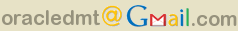## Matrix Inversion Using PL/SQL

Recently someone asked me how to invert a matrix using the UTL_NLA PL/SQL package. This can be done by solving a system of linear equations AX = B like the ones I described in this post but setting B to the identity matrix. I thought that the question was interesting enough to deserve its own post. So I am replicating the answer here for those interested.

The UTL_NLA package has many different procedures for solving systems of linear equations. Most of them are designed to take advantage of special form matrices (e.g., triangular, banded, etc.). For the example below, I picked the procedure LAPACK_GELS. This procedure solves general systems of linear equations in a robust fashion. It uses QR or LQ decompositions as needed.

```DECLARE
A     utl_nla_array_dbl :=  utl_nla_array_dbl(
5,   153,  352,
153, 5899, 9697,
352, 9697, 26086);
B     utl_nla_array_dbl := utl_nla_array_dbl(
1, 0, 0,
0, 1, 0,
0, 0, 1);
ipiv  utl_nla_array_int := utl_nla_array_int(0,0);
info  integer;
n     NUMBER := 3;
i     NUMBER;
j     NUMBER;
BEGIN

UTL_NLA.LAPACK_GELS (
trans  => 'N',  -- solve for A instead of A'
m      => n,    -- A number of rows
n      => n,    -- A number of columns
nrhs   => n,    -- B number of columns
a      => A,    -- matrix A
lda    => n,    -- max(1, m)
b      => B,    -- matrix B
ldb    => n,    -- ldb >= max(1, m, n)
info   => info, -- operation status (0=sucess)
pack   => 'R'   -- how the matrices are stored
-- (C=column-wise)
);

dbms_output.put_line('inv(A)');
dbms_output.put_line('-------------');
dbms_output.put_line('info= '||info);
IF info=0 THEN
FOR l IN 1..B.count LOOP
i := ceil(l/n);
j := l-(i-1)*n;
dbms_output.put_line('x[' || i ||']['||j||']= ' ||
TO_CHAR(B(l),'99.9999'));
END LOOP;
END IF;
END;
/```
Here is the output for the above code:
```inv(A)
-------------
info= 0
x=  27.5307
x=   -.2658
x=   -.2727
x=   -.2658
x=    .0030
x=    .0025
x=   -.2727
x=    .0025
x=    .0028```

Labels: ,

### var a = 5; if(a == 0) {document.write('No comment');} else if(a == 1) {document.write('1 comment');}else{document.write(a+' comments');}

Very nice.

Good to see you are back to posting.

Mathew Butler

In the AX = B form of the system of equations isn't B supposed be a vector rather than a square matrix?

If A is n x n and X is n x 1 [n rows, 1 column] then B has to be n x 1 too.

In my previous examples in another post X was a vector. However, in the equation AX=B, X does not have to be a vector. In this example, we want X to be the inverse of A. So, if A is n x n then X=inv(A) is also n x n and B is the n x n identity matrix.

Sorry to be a pain but have you ever managed to extend this to calculate the generalized inverse of an nxn matrix?

Sorry to be a pain but have you ever extended this to calculate the generalized inverse of an nxn matrix?

• Marcos M. Campos: Development Manager for Oracle Data Mining Technologies. Previously Senior Scientist with Thinking Machines. Over the years I have been working on transforming databases into easy to use analytical servers.
• My profileAll Posts

## Category Cloud

• Business intelligence• Data mining• Oracle analytics